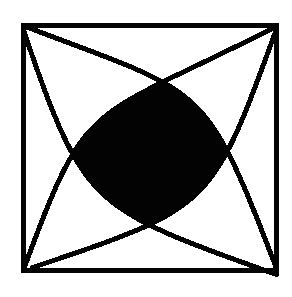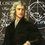# Tricky!How shall I find the area of shaded region?

It is given that the figure is a square with each side as 14 cm. and taking each side of the square as radius 4 quadrants are drawn find the area of the shaded region.Note by Avn Bha
6 years, 4 months ago

This discussion board is a place to discuss our Daily Challenges and the math and science related to those challenges. Explanations are more than just a solution — they should explain the steps and thinking strategies that you used to obtain the solution. Comments should further the discussion of math and science.

When posting on Brilliant:

• Use the emojis to react to an explanation, whether you're congratulating a job well done , or just really confused .
• Ask specific questions about the challenge or the steps in somebody's explanation. Well-posed questions can add a lot to the discussion, but posting "I don't understand!" doesn't help anyone.
• Try to contribute something new to the discussion, whether it is an extension, generalization or other idea related to the challenge.
• Stay on topic — we're all here to learn more about math and science, not to hear about your favorite get-rich-quick scheme or current world events.

MarkdownAppears as
*italics* or _italics_ italics
**bold** or __bold__ bold
- bulleted- list
• bulleted
• list
1. numbered2. list
1. numbered
2. list
Note: you must add a full line of space before and after lists for them to show up correctly
paragraph 1paragraph 2

paragraph 1

paragraph 2

[example link](https://brilliant.org)example link
> This is a quote
This is a quote
    # I indented these lines
# 4 spaces, and now they show
# up as a code block.

print "hello world"
# I indented these lines
# 4 spaces, and now they show
# up as a code block.

print "hello world"
MathAppears as
Remember to wrap math in $$ ... $$ or $ ... $ to ensure proper formatting.
2 \times 3 $2 \times 3$
2^{34} $2^{34}$
a_{i-1} $a_{i-1}$
\frac{2}{3} $\frac{2}{3}$
\sqrt{2} $\sqrt{2}$
\sum_{i=1}^3 $\sum_{i=1}^3$
\sin \theta $\sin \theta$
\boxed{123} $\boxed{123}$

## Comments

Sort by:

Top Newest

Here's my solution:soln

- 6 years, 4 months ago

Log in to reply

it is much simpler and sauber.thanks

- 6 years, 4 months ago

Log in to replyI suggest to find the area $A = 4 A_1 + A_2$, where:

• $A_1$ is the area of the green chord
• $A_2$ is the area of yellow square

Since $\triangle ADQ$ and $\triangle PDC$ are equilateral triangles, $\angle PDQ$ is $\space 30^\circ$. Let $R= 14$ cm, then we have:

$A_1 = \text {Area of segment DPQ} - \text {Area of } \triangle DPQ = \dfrac {30^\circ}{360^\circ} \pi R^2 - \frac {1}{2} (2R \cos{75^\circ} )(R \sin {75^\circ} )$

$\quad \space = \dfrac {\pi R^2}{12} - R^2 \sin {75^\circ} \cos {75^\circ} = \left( \dfrac {\pi}{12} - \dfrac {1}{2} \sin {150^\circ} \right) R^2 = \left( \dfrac {\pi}{12} - \dfrac {1}{4} \right) R^2$

$A_2 = (2R\cos {75^\circ})^2 = 4R^2 \cos^2 {75^\circ} = (2\cos {150^\circ} + 2)R^2 = (2-\sqrt{3})R^2$

Therefore, $A = 4A_1+A_2 = 4\left( \dfrac {\pi}{12} - \dfrac {1}{4} \right) R^2 + (2-\sqrt{3})R^2 = \left( \dfrac {\pi}{3} + 1 -\sqrt{3} \right) R^2 = \boxed {61.76876175}$

- 6 years, 4 months ago

Log in to reply

Novel approach!

- 6 years, 4 months ago

Log in to reply

Whose diagram are you referencing?

- 6 years, 4 months ago

Log in to reply

Sorry, the diagram did not turn out well earlier.

- 6 years, 4 months ago

Log in to reply

could we use coordinate geometry to find the points of intersection and then use integration?

- 6 years, 4 months ago

Log in to reply

My first thought was to do just that, but then I came up with a purely geometric approach, which is probably what Avn is after.

- 6 years, 4 months ago

Log in to reply

I would divide the shaded area up into four subregions of equal area, the dividing lines being the line segments joining the midpoints of directly opposite sides of the square. Let the origin be the lower left corner of the square, and focus on the subregion furthest from the origin. Now form a sector formed by the origin and the arc of this subregion. This sector has a central angle of $30^{\circ}$ and sides length $14$. Now form two triangles joining the origin to the lower left corner of the subregion and to one of the endpoints of the arc described above. The area of the subregion will then be the area of the sector minus the areas of these two triangles.

Each of these triangles has side lengths $14$ and $7\sqrt{2}$ with an angle of $15^{\circ}$ between them. The area of each is then $(\frac{1}{2})(14)(7\sqrt{2})\sin(15^{\circ}) = (\frac{49}{2})(\sqrt{3} - 1)$. The area of the sector is $(\frac{49}{3})\pi$, and thus the area of the subregion is

$(\frac{49}{3})\pi - 49(\sqrt{3} - 1) = (\frac{49}{3})(\pi + 3 - 3\sqrt{3})$.

The area of the entire shaded region is then just four times the area of the subregion, giving an answer of

$\dfrac{196}{3} * (\pi + 3 - 3\sqrt{3}) = 61.769$ to $3$ decimal places.

This represents an area of about $31.5$% of that of the square. When the quarter-circles are drawn more accurately, this appears to the eye as a reasonable percentage coverage.

- 6 years, 4 months ago

Log in to reply

I often prefer the geometric approach, but that one was already done. So here is the calculus approach for your viewing pleasure.

First, given the nature of this figure, it's symmetrical and can be split into 4 equal parts by connecting the intersections with a vertical and horizontal line (symmetric figures are nice like that). The calculus portion focuses on the upper-right portion.

To write three of the curves as functions:

Upper-right circle: $f(x)=\sqrt{14^{2}-x^{2}}$

Upper-left circle: $g(x)=\sqrt{14^{2}-(x-14)^{2}}$

Lower-right circle: $h(x)=14-\sqrt{14^{2}-x^{2}}$

Staying in the domain and range of $[0,14]$ , if you set $f(x)=g(x)$ you'll get $(7,7\sqrt{3})$ , and from $f(x)=h(x)$ you'll get $(7\sqrt{3},7)$.

Four times the area of the upper-right portion represents the area of the shaded regions, and is represented with:

$4\int_{7}^{7\sqrt{3}}( \sqrt{14^{2}-x^{2}}-7)dx = 4\int_{7}^{7\sqrt{3}}( \sqrt{14^{2}-x^{2}})dx-28\int_{7}^{7\sqrt{3}}dx$

The second half of that is $28(7\sqrt{3}-7) = 196(\sqrt{3}-1)$.

For the first half, use the following trig substitutions:

$x = 14\sin u$

$dx = 14\cos udu$

$x=7 \Rightarrow u=\sin^{-1}\frac{1}{2} = \frac{\pi}{6}$

$x=7\sqrt{3} \Rightarrow u=\sin^{-1}\frac{\sqrt{3}}{2} = \frac{\pi}{3}$

Which turns the first half of the integral into:

$\Rightarrow 4\int_{\frac{\pi}{6}}^{\frac{\pi}{3}}( \sqrt{14^{2}-14^{2}\sin^{2}u})(14\cos u)du=4\int_{\frac{\pi}{6}}^{\frac{\pi}{3}}( \sqrt{14^{2}(1-\sin^{2}u}))(14\cos u)du$

$=4\int_{\frac{\pi}{6}}^{\frac{\pi}{3}}( \sqrt{14^{2}\cos^{2}u})(14\cos u)du=784\int_{\frac{\pi}{6}}^{\frac{\pi}{3}}( \cos^{2}u)du=392\int_{\frac{\pi}{6}}^{\frac{\pi}{3}}(1+\cos 2u)du$

$=392(u+\frac{1}{2}\sin 2u)|_\frac{\pi}{6}^\frac{\pi}{3}=196(\frac{\pi}{3})$

The first part minus the second part gives $196(\frac{\pi}{3}) - 196(\sqrt{3}-1) = \boxed{196(\frac{\pi}{3}-\sqrt{3}+1)}$.

- 6 years, 4 months ago

Log in to reply

You can make an algorithm count the number of black pixels within the drawing. Then calculate the percentage of pixels within the shape out of the pixels that are within the square and multiply by 14^2.

- 6 years, 4 months ago

Log in to reply

×

Problem Loading...

Note Loading...

Set Loading...